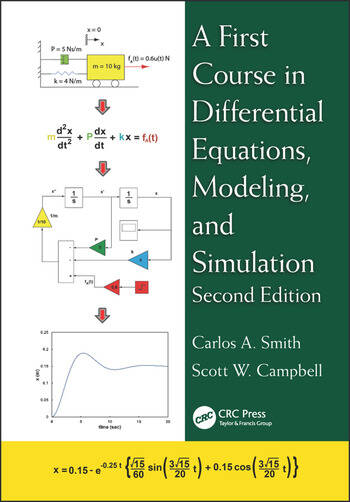# A First Course in Differential Equations, Modeling, and Simulation

## 2nd Edition

Carlos A. Smith, Scott W. Campbell

CRC Press
Published February 5, 2016
Textbook - 529 Pages - 320 B/W Illustrations
ISBN 9781482257229 - CAT# K24086

For Instructors Request Inspection Copy

USD\$110.00

FREE Standard Shipping!

## Preview

### Summary

A First Course in Differential Equations, Modeling, and Simulation shows how differential equations arise from applying basic physical principles and experimental observations to engineering systems. Avoiding overly theoretical explanations, the textbook also discusses classical and Laplace transform methods for obtaining the analytical solution of differential equations. In addition, the authors explain how to solve sets of differential equations where analytical solutions cannot easily be obtained.

Incorporating valuable suggestions from mathematicians and mathematics professors, the Second Edition:

• Expands the chapter on classical solutions of ordinary linear differential equations to include additional methods
• Increases coverage of response of first- and second-order systems to a full, stand-alone chapter to emphasize its importance
• Includes new examples of applications related to chemical reactions, environmental engineering, biomedical engineering, and biotechnology
• Contains new exercises that can be used as projects and answers to many of the end-of-chapter problems
• Features new end-of-chapter problems and updates throughout

Thus, A First Course in Differential Equations, Modeling, and Simulation, Second Edition provides students with a practical understanding of how to apply differential equations in modern engineering and science.

#### Instructors

We provide complimentary e-inspection copies of primary textbooks to instructors considering our books for course adoption.